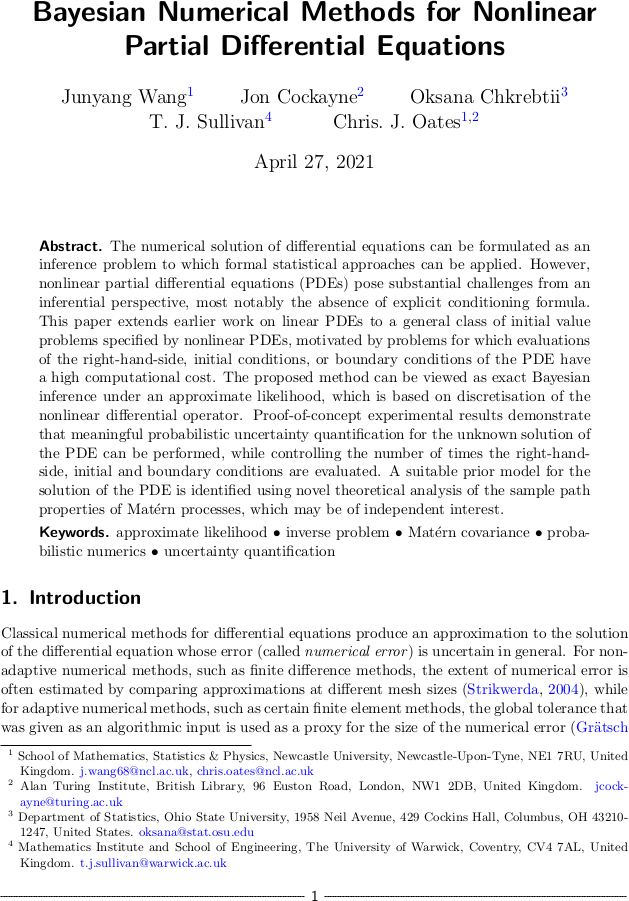# Tim Sullivan

### #chkrebtii

Clear Search### Bayesian numerical methods for nonlinear PDEs

Junyang Wang, Jon Cockayne, Oksana Chkrebtii, Chris Oates, and I have just uploaded a preprint of our recent work “Bayesian numerical methods for nonlinear partial differential equations” to the arXiv. This paper continues our study of (approximate) Bayesian probabilistic numerical methods (Cockayne et al., 2019), in this case for the challenging setting of nonlinear PDEs, with the goal of realising a posterior distribution over the solution of the PDE that carries a meaningful expression of uncertainty about the solution's true value given the discretisation error that has been incurred.

Abstract. The numerical solution of differential equations can be formulated as an inference problem to which formal statistical approaches can be applied. However, nonlinear partial differential equations (PDEs) pose substantial challenges from an inferential perspective, most notably the absence of explicit conditioning formula. This paper extends earlier work on linear PDEs to a general class of initial value problems specified by nonlinear PDEs, motivated by problems for which evaluations of the right-hand-side, initial conditions, or boundary conditions of the PDE have a high computational cost. The proposed method can be viewed as exact Bayesian inference under an approximate likelihood, which is based on discretisation of the nonlinear differential operator. Proof-of-concept experimental results demonstrate that meaningful probabilistic uncertainty quantification for the unknown solution of the PDE can be performed, while controlling the number of times the right-hand-side, initial and boundary conditions are evaluated. A suitable prior model for the solution of the PDE is identified using novel theoretical analysis of the sample path properties of Matérn processes, which may be of independent interest.

Published on Tuesday 27 April 2021 at 10:00 UTC #preprint #prob-num #wang #cockayne #chkrebtii #oates